GATE  >  Test : CSE Past Year Paper 2020

# Test : CSE Past Year Paper 2020

Test Description

## 65 Questions MCQ Test | Test : CSE Past Year Paper 2020

Test : CSE Past Year Paper 2020 for GATE 2023 is part of GATE preparation. The Test : CSE Past Year Paper 2020 questions and answers have been prepared according to the GATE exam syllabus.The Test : CSE Past Year Paper 2020 MCQs are made for GATE 2023 Exam. Find important definitions, questions, notes, meanings, examples, exercises, MCQs and online tests for Test : CSE Past Year Paper 2020 below.
Solutions of Test : CSE Past Year Paper 2020 questions in English are available as part of our course for GATE & Test : CSE Past Year Paper 2020 solutions in Hindi for GATE course. Download more important topics, notes, lectures and mock test series for GATE Exam by signing up for free. Attempt Test : CSE Past Year Paper 2020 | 65 questions in 180 minutes | Mock test for GATE preparation | Free important questions MCQ to study for GATE Exam | Download free PDF with solutions
 1 Crore+ students have signed up on EduRev. Have you?
Test : CSE Past Year Paper 2020 - Question 1

### Goods and Services Tax (GST) is an indirect tax introduced in India in 2017 that is imposed on the supply of goods and services, and it subsumes all indirect taxes except few. It is a destination-based tax imposed on goods and services used, and it is not imposed at the point of origin from where goods come. GST also has a few components specific to state governments, central government and Union Territories (UTs). Which one of the following statements can be inferred from the given passage?

Detailed Solution for Test : CSE Past Year Paper 2020 - Question 1

According to passage GST is imposed on the supply of goods and services hence option (a) is incorrect which states that GST is imposed on production of goods and services.
In the passage it is mentioned that GST has a two components specific to UTs. So option (b) is incorrect.
Passage states that GST is a destination based tax which means it is imposed at the point of usage of goods and services. Hence (c) is correct.
Option (d) says GST includes all indirect taxes but as per passage it subsumes all indirect taxes except few.

Test : CSE Past Year Paper 2020 - Question 2

### Raman is confident of speaking English ________ six months as he has been practising regularly _________ the last three weeks.

Detailed Solution for Test : CSE Past Year Paper 2020 - Question 2

• ‘within’ is a preposition that is used to express something that occurs inside a particular period of time.
• ‘for’ is used here because
(i) Sentence is in ‘present perfect continuous tense’.
(ii) For is used when we talk about a period of time.

Test : CSE Past Year Paper 2020 - Question 3

### If P = 3, R = 27, T = 243, then Q + S = ________.

Detailed Solution for Test : CSE Past Year Paper 2020 - Question 3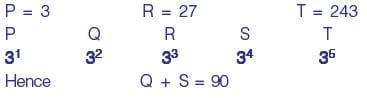Test : CSE Past Year Paper 2020 - Question 4

Two straight lines are drawn perpendicular to each other in X-Y plane. If α and β are the acute angles the straight lines make with the X-axis, then α + β is ________.

Test : CSE Past Year Paper 2020 - Question 5

The figure below shows an annular ring with outer and inner radii as b and a, respectively.
The annular space has been painted in the form of blue colour circles touching the outer and inner periphery of annular space. If maximum n number of circles can be painted, then the unpainted are available in annular space is ________.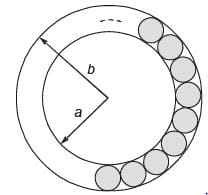Detailed Solution for Test : CSE Past Year Paper 2020 - Question 5

Unpainted area = Area of annular ring – Area of n blue color circles
Area of annular ring = πb2 – πa2
i.e. π(b2 – a2)
Area of 1 blue circle =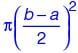Hence, area of n blue circles =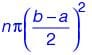⇒ Unpainted area =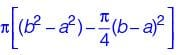Test : CSE Past Year Paper 2020 - Question 6

There are multiple routes to reach from node 1 to node 2, as shown in the network.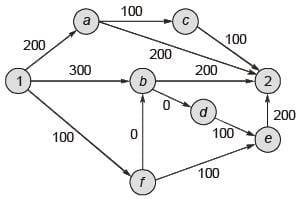The cost of travel on an edge between two nodes is given in rupees. Nodes ‘a’ ‘b’, ‘c’, ‘d’, ‘e’ and ‘f’ are toll booths. The toll price at toll booths marked ‘a’ and ‘c’ is Rs. 200. and is Rs. 100 for the other toll booths. Which is the cheapest route from node 1 to node 2?

Detailed Solution for Test : CSE Past Year Paper 2020 - Question 6

• Cost of 1-a-C-2 : 200 + 200 + 100 + 100 + 100 = 700
• Cost of 1-b-2 : 300 + 100 + 200 = 600
• Cost of 1-f-b-2 : 100 + 100 + 100 + 200 = 500
• Cost of 1-f-e-2 :100 + 100 + 100 + 200 + 200 = 700
Hence, 1-f-b-2 is having minimum cost.

Test : CSE Past Year Paper 2020 - Question 7

His knowledge of the subject was excellent but his classroom performance was ________.

Detailed Solution for Test : CSE Past Year Paper 2020 - Question 7

‘But’ is used for introducing an idea which contrasts with the statement that has been already said.

Test : CSE Past Year Paper 2020 - Question 8

Select the word that fits the analogy:
Cook : Cook :: Fly : ________

Detailed Solution for Test : CSE Past Year Paper 2020 - Question 8

(i) Relation is verb : noun
(ii) One who cooks is a cook, similarly one who flies any aircraft is a flyer.

Test : CSE Past Year Paper 2020 - Question 9

The dawn of the 21st century witnessed the melting glaciers oscillating between giving too much and too little to billions of people who depend on their for fresh water. The UN climate report estimates that without deep cuts to man-made emissions, at least 30% of the northern hemisphere’s surface permafrost could melt by the end of the century.Given this situation of imminent global exodus of billions of people displaced by rising seas, nation-states need to rethink their carbon footprint for political concerns, if not for environmental ones.
Which one of the following statements can be inferred from the given passage?

Test : CSE Past Year Paper 2020 - Question 10

The total revenue of a company during 2014-2018 is shown in the bar graph. If the total expenditure of the company in each year is 500 million rupees, then the aggregate profit loss (in percentage) on the total expenditure of the company during 2014-2018 is ________.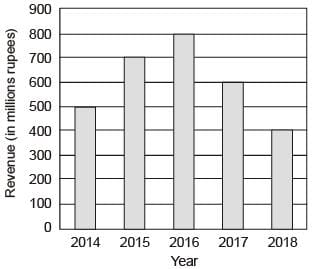Detailed Solution for Test : CSE Past Year Paper 2020 - Question 10

Total expenditure = 2500 million
Total revenue = 3000 million
So, profit %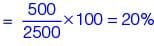*Answer can only contain numeric values
Test : CSE Past Year Paper 2020 - Question 11

Let R be the set of all binary relations on the set {1, 2, 3}. Suppose a relation is chosen from R at random. The probability that the chosen relation is reflexive (round off to 3 decimal places) is ________.

Detailed Solution for Test : CSE Past Year Paper 2020 - Question 11

A = {1, 2, 3}
n = ⏐A⏐ = 3
Number of relations on A =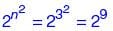Number of reflexive relations on A =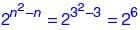P(reflexive relation) =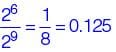Test : CSE Past Year Paper 2020 - Question 12

Consider a relational database containing the following schemas.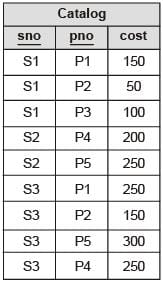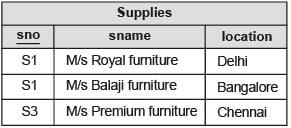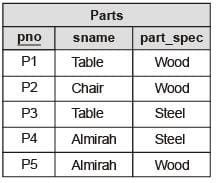The primary key of each table is indicated by underlining the constituent fields.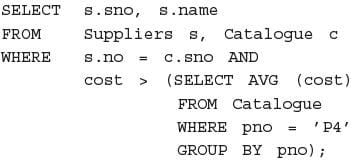The number of rows returned by the above SQL query is

Detailed Solution for Test : CSE Past Year Paper 2020 - Question 12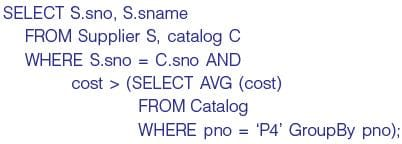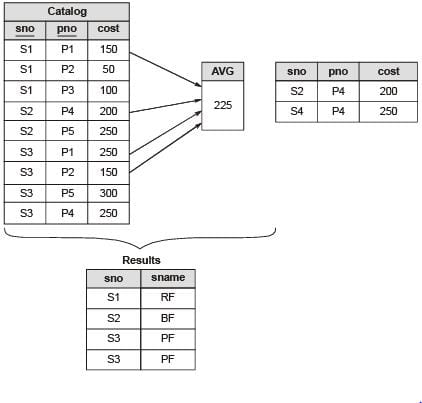∴ 4 rows in table.

Test : CSE Past Year Paper 2020 - Question 13

Which one of the following is used to represent the supporting many-ore relationships of a weak entity set in an entity-relationship diagram?

Detailed Solution for Test : CSE Past Year Paper 2020 - Question 13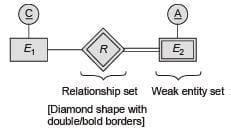Test : CSE Past Year Paper 2020 - Question 14

Consider allocation of memory to a new process. Assume that none of the existing holes in the memory will exactly fit the process’s memory requirement. Hence, a new hole of smaller size will be created if allocation is made in any of the existing holes. Which one of the following statements is TRUE?

Detailed Solution for Test : CSE Past Year Paper 2020 - Question 14

The hole created by best fit is never larger than the hole created by first fit. This is correct option.

Test : CSE Past Year Paper 2020 - Question 15

What is the worst case time complexity of inserting n elements into an empty linked list, if the linked list needs to be maintained in sorted order?

Detailed Solution for Test : CSE Past Year Paper 2020 - Question 15

Insert element at the beginning of linked list, take Ο(1)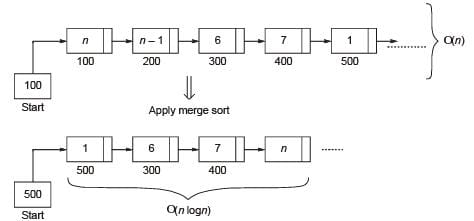*Answer can only contain numeric values
Test : CSE Past Year Paper 2020 - Question 16

Consider the following C program: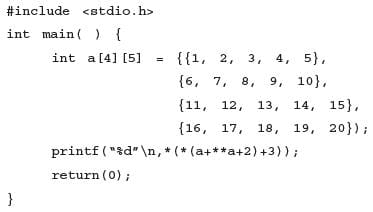The output of the program is ________.

Test : CSE Past Year Paper 2020 - Question 17

Consider the functions:
I. e-x
II. x2 – sin x
III.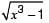Which of the above functions is/are increasing everywhere in [0,1]?

Detailed Solution for Test : CSE Past Year Paper 2020 - Question 17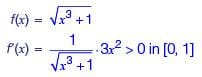∴ Hence it is increasing function.

Test : CSE Past Year Paper 2020 - Question 18

Consider the following statements about process state transitions for a system using preemptive scheduling.
I. A running process can move to ready state.
II. A ready process can move to running state.
III. A blocked process can move to running state.
IV. A blocked process can move to ready state.
Which of the above statements arc TRUE?

Detailed Solution for Test : CSE Past Year Paper 2020 - Question 18

Statement I, II and IV are correct.

Test : CSE Past Year Paper 2020 - Question 19

Consider the following statements:
I. If L1 ∪ L2 is regular, then both L1 and L2 must be regular.
II. The class of regular languages is closed under infinite union.
Which of the above statements is/are TRUE?

Detailed Solution for Test : CSE Past Year Paper 2020 - Question 19

• If L1 ∪ L2 is regular, then neither needs to be regular.
Example: {anbn} ∪ {anbn}c = (a + b)* is regular but {anbn} and its complement both are non-regular.
So statement I is false.
• The class of regular language is not closed under infinite union.
Proof:  It is was closed under infinite union then
anbn = {∈} ∪ {ab } ∪ {aabb } ∪ ........ will be infinite union of finite languages (which are regular) and hence will become regular. But we know that {anbn⏐n ≥ 0} is nonregular.
So II is false
So option (a), neither I nor II is the correct answer.

Test : CSE Past Year Paper 2020 - Question 20

The preorder traversal of a binary search tree is 15, 10. 12, 11, 20, 18,  16, 19. Which one of the following is the postorder traversal of the tree?

Detailed Solution for Test : CSE Past Year Paper 2020 - Question 20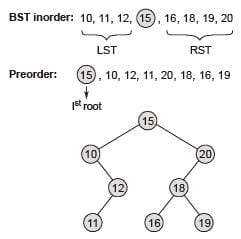Postorder: 11, 12, 10, 16, 19, 18, 20, 15

*Answer can only contain numeric values
Test : CSE Past Year Paper 2020 - Question 21

Consider the following grammar:
S → aSB⏐d
B → b
The number of reduction steps taken by a bottom-up parser while accepting the string aaadbbb is ________.

Detailed Solution for Test : CSE Past Year Paper 2020 - Question 21

S → aSB
→ aaSBB [S → aSB]
→ aaaSBBB [S → aSB]
Total 7 steps required.

Test : CSE Past Year Paper 2020 - Question 22

Which one of the following regular expressions represents the set of all binary strings with an odd number of 1’s?

Detailed Solution for Test : CSE Past Year Paper 2020 - Question 22

• Regular expression in option (a) is incorrect because it will force the strings to end with 1 and a string of odd number of 1’s need not to end with 1.
• Regular expression in option (b) will force it to start with 1 and hence it is incorrect.
• Regular expression in option (c) can create odd number of 1’s as well as even number of 1’s and hence it is incorrect.
• Regular expression in option (d) is incorrect as it does not generate strings ‘01’ or 1 or more 0 followed by 1 which is having an odd number of 1’s.
Note: Option (d) would be correct only when if the expression were (0*10*10*)*10* + (0*10*) means (0*10*) missing from the option. Hence option (d) is also incorrect.

Test : CSE Past Year Paper 2020 - Question 23

What is the worst case time complexity of inserting n2 elements into an AVL-tree with n elements initially?

Detailed Solution for Test : CSE Past Year Paper 2020 - Question 23

AVL with n element: [height balanced [–1, 0, +1] BST]
logn level due to balanced BST.
(i) Every insertion of element:
logn: Find place to insert.
logn: If property not satisfied do rotation.
∴ n2 element insertion:
For 1 element ≡ 2 logn
So, for n2 element ≡ θ(n2 logn)

Test : CSE Past Year Paper 2020 - Question 24

Consider the language L = {an⏐n > 0} ∪ {anbn⏐n ≥ 0} and the following statements.
I. L is deterministic context-free.
II. L is context-free but not deterministic context-free.
III. L is not LL(k ) for any k.
Which of the above statements is/are TRUE?

Detailed Solution for Test : CSE Past Year Paper 2020 - Question 24

• Statement I: L in DCFL is true, since {an} ∪ {anbn} = regular ∪ DCFL = DCFL, by closure property. So II is false and I is true.
• Statement III is true because we cannot write LL(k ) grammar, for any value of k , since no matter how many a’s are shown to compiler it will be impossible to distinguish between whether the string presented is in the form of {an} or {anbn} and hence the production cannot be chosen uniquely for any value of k.
So option (a) is correct, I and III only are true.

*Answer can only contain numeric values
Test : CSE Past Year Paper 2020 - Question 25

A multiplexer is placed between a group of 32 registers and an accumulator to regulate data movement such that at any given point in time the content of only one register will move to the accumulator, The minimum number of select lines needed for the multiplexer is _________.

Detailed Solution for Test : CSE Past Year Paper 2020 - Question 25

Number of registers = n = 32
Required multiplexer size is n : 1 i.e. 32 : 1
No of select lines required to the multiplexer = m
∴ m = log2 n
m = log2 32
m = 5

*Answer can only contain numeric values
Test : CSE Past Year Paper 2020 - Question 26

If there are m input lines and n output lines for a decoder that is used to uniquely address a byte addressable 1 KB RAM, then the minimum value of m + n is ________.

Detailed Solution for Test : CSE Past Year Paper 2020 - Question 26

We need 210 outputs to map 1 KB RAM.
For this we need 10 × 210 decoder.
Here m = 10 and n = 210
m + n = 1034

*Answer can only contain numeric values
Test : CSE Past Year Paper 2020 - Question 27

A direct mapped cache memory of 1 MB has a block size of 256 bytes. The cache has an access time of 3 ns and a hit rate of 94%. During a cache miss, it takes 20 ns to bring the first word of a block from the main memory, while each subsequent word takes 5 ns. The word size is 64 bits. The average memory access time in ns (round off to 1 decimal place) is ________.

Detailed Solution for Test : CSE Past Year Paper 2020 - Question 27

Word size = 64 bit = 8B
Block size = 256B
∴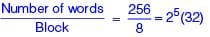Tavg = (0.94 × 3) + (1 – 0.94) [3 + 20 + (31 × 5)]
= 13.5 ns

*Answer can only contain numeric values
Test : CSE Past Year Paper 2020 - Question 28

Consider a double hashing scheme in which the primary hash function is h1(k) = k mod 23 and the secondary hash function is h2(k) = 1 + (k mod 19). Assume that the table size is 23. Then the address returned by probe 1 in the probe sequence (assume that the probe sequence begins at probe 0) for key value k = 90 is ________.

Detailed Solution for Test : CSE Past Year Paper 2020 - Question 28

For double hashing we use the formula as (h1(k) + i h2(k ))% table size where i denotes probe value.
h1(k) = 90% 23 = 21
h2(k) = 1 + k % 19
= 1 + 90 % 19 = 15
For probe 1 the value of i is 1 thus, (21 + 13) % 23 = 13

Test : CSE Past Year Paper 2020 - Question 29

Consider the following statements about the functionality of an IP based router.
I. A router does not modify the IP packets during forwarding.
II. It is not necessary for a router to implement any routing protocol.
III. A router should reassemble IP fragments if the MTU of the outgoing link is larger than the size of the incoming IP packet.
Which of the above statements is/are TRUE?

Detailed Solution for Test : CSE Past Year Paper 2020 - Question 29

I. A router modifies the IP packets during forwarding because TTL is changing.
II. A router can also be used in LAN network it does not require routing protocol at that time.
III. Packet fragmentation is done if packet size is more than MTU.

Test : CSE Past Year Paper 2020 - Question 30

Consider the following data path diagram.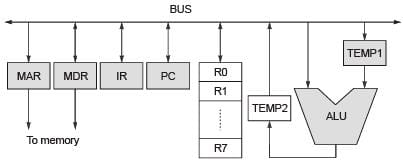Consider an instruction: R0 ← R1 + R2. The following steps are used to execute it over the given data path. Assume that PC is incremented appropriately. The subscripts r and w indicate read and write operations, respectively.
2. R1r, TEMP1w
3. PCr, MARw, MEMr
4. TEMP2r, R0w
5. MDRr, IRw
Which one of the following is the correct order of execution of the above steps?

Detailed Solution for Test : CSE Past Year Paper 2020 - Question 30

1. Send the address to memory via MAR.
2. Read the opcode into IR from the memory via MBR.
3. Send the first operand to Temp1(ALU).
4. Read the second operand directly from the R2 and process the data in ALU and store the result into TEMP2.
5. Store the result into R0.

*Answer can only contain numeric values
Test : CSE Past Year Paper 2020 - Question 31

Assume that you have made a request for a web page through your web browser to a web server. Initially the browser cache is empty. Further, the browser is configured to send HTTP requests in non-persistent mode. The web page contains text and five very small images. The minimum number of TCP connections required to display the web page completely in your browser is ________.

Detailed Solution for Test : CSE Past Year Paper 2020 - Question 31

In non persistent HTTP for every objects there is a TCP connection required.
Hence 1 TCP connection for text and 5 TCP connections for images required.
So total 6 connections are required.

*Answer can only contain numeric values
Test : CSE Past Year Paper 2020 - Question 32

Let G be a group of 35 elements. Then the largest possible size of a subgroup of G other than G itself is ________.

Detailed Solution for Test : CSE Past Year Paper 2020 - Question 32

Size of group = Ο(G) = 35
Lagranges theorem says if H is subgroup of G then Ο(H)⏐Ο(G).
So Ο(H) can be 1, 5, 7 or 35.
So largest subgroup size other than G itself is 7.

Test : CSE Past Year Paper 2020 - Question 33

Consider the following statements:
I. Daisy chaining is used to assign priorities in attending interrupts.
II. When a device raises a vectored interrupt, the CPU does polling to identify the source of interrupt.
III. In polling, the CPU periodically checks the status bits to know if any device needs its attention.
IV. During DMA, both the CPU and DMA controller can be bus masters at the same time.
Which of the above statements is/are TRUE?

Test : CSE Past Year Paper 2020 - Question 34

For parameters a and b, both of which are ω(1), T (n) = T (n1/a) + 1, and T (b) = 1. Then T (n) is

Test : CSE Past Year Paper 2020 - Question 35

Consider the following statements:
I. Symbol table is accessed only during lexical analysis and syntax analysis.
II. Compilers for programming languages that support recursion necessarily need heap storage for memory allocation in the run-time environment.
III. Errors violating the condition ‘any variable must be declared before its use ’ are detected during syntax analysis.
Which of the above statements is/are TRUE?

Detailed Solution for Test : CSE Past Year Paper 2020 - Question 35

I. False. Symbol Table is used by all 6 phases of compiler: lexical analysis, syntax analysis, semantic analysis, intermediate code generation, code optimization, and target code generation phase.

II. False. Heap allocation is used to dynamically allocate memory to the variables. Heap allocation can be used for recursion, but recursion is also supported in Stack allocation.

III. False. Syntax analysis checks only syntax, but variable declare before its use must be done by Semantic analyses.

None of the given statements are correct.

*Answer can only contain numeric values
Test : CSE Past Year Paper 2020 - Question 36

Consider the array representation of a binary min-heap containing 1023 elements. The minimum number of comparisons required to find the maximum in the heap ________.

Detailed Solution for Test : CSE Past Year Paper 2020 - Question 36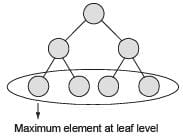Number of element = [1023/2] = 512
Now apply bubble sort requires 511 comparisons.

*Answer can only contain numeric values
Test : CSE Past Year Paper 2020 - Question 37

Consider a paging system that uses 1-level page table residing in main memory and a TLB for address translation. Each main memory access takes 100 ns and TLB lookup takes 20 ns. Each page transfer to/from the disk takes 5000 ns. Assume that the TLB hit ratio is 95%, page fault rate is 10%. Assume that for 20% of the total page faults, a dirty page has to be written back to disk before the required page is read in from disk. TLB update time is negligible. The average memory access time in ns (round off to 1 decimal places) is ________.

Detailed Solution for Test : CSE Past Year Paper 2020 - Question 37

EMAT = 0.95 × (20 + 100) + 0.05 × (0.9 × (20 + 100 + 100) + 0.1 × [0.2 ×
(20 + 100 + 5000 + 5000) + 0.8 × (20 + 100 + 5000)] = 154.5 ns

Test : CSE Past Year Paper 2020 - Question 38

Each of a set of n processes executes the following code using two semaphores a and b initialized to 1 and 0, respectively. Assume that count is a shared variable initialized to 0 and not used in CODE SECTION P.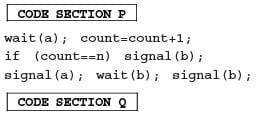What does the code achieve?

Detailed Solution for Test : CSE Past Year Paper 2020 - Question 38

All the processes running the given code will remain block due to wait(b) until the value of count becomes n.
When the value of count will become equals to n, value of b changes to 1 which will subsequently allow a process to go into section Q.
Hence, no process can go to section Q until all the process executes code section P.

Test : CSE Past Year Paper 2020 - Question 39

Let G = (V, E ) be a weighted undirected graph and let T be a Minimum Spanning Tree (MST) of G maintained using adjacency lists. Suppose a new weighted edge (u, v ) ∈ V × V is added to G. The worst case time complexity of determining if T is still an MST of the resultant graph is

Test : CSE Past Year Paper 2020 - Question 40

Consider the following five disk access requests of the form (request id, cylinder number) that are present in the disk scheduler queue at a given time.
(P, 155), (Q, 85), (R, 110), (S, 30), (T, 115)
Assume the head is positioned at cylinder 100. The scheduler follows Shortest Seek Time First scheduling to service the requests,
Which one of the following statements is FALSE?

*Answer can only contain numeric values
Test : CSE Past Year Paper 2020 - Question 41

Consider the following language:
L = {x ∈ {a, b}*⏐number of a’s in x is divisible by 2 but not divisible by 3} The minimum number of states in a DFA that accepts L is ________.

Detailed Solution for Test : CSE Past Year Paper 2020 - Question 41

The minimum number of states in a DFA that accepts:
L = { x ∈ {a, b }*⏐ number of a’s is divisible by 2 but not divisible by 3} is 6 states as shown below: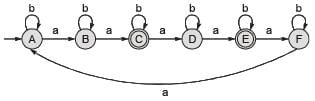(or) alternatively  it can be designed by taking a product automata of
L1 = {x ∈ {a, b }*⏐ number of a’s divisible by 2} and L2 = { x ∈ {a , b }*⏐ number of a’s not divisible by 3} as shown below:
Minimum DFA for L1: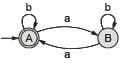Minimum DFA for L2: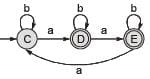Product automata L1 ∩ L2 having six states, shown below: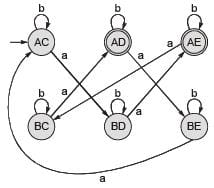Which is same as our directly designed machine with 6 states.

*Answer can only contain numeric values
Test : CSE Past Year Paper 2020 - Question 42

Consider the following C functions: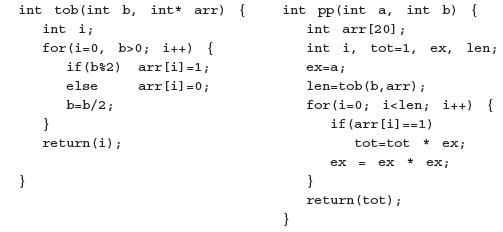The value returned by pp(3,4) is ________.

Detailed Solution for Test : CSE Past Year Paper 2020 - Question 42

After executing the given C program integer 81 is returned.

*Answer can only contain numeric values
Test : CSE Past Year Paper 2020 - Question 43

Consider a non-pipelined processor operating at 2.5 GHz. It takes 5 clock cycles to complete an instruction. You are going to make a 5-stage pipeline out of this processor.
Overheads associated with pipelining force you to operate the pipelined processor at 2 GHz. In a given program, assume that 30% are memory instructions. 60% are ALU instructions and the rest are branch instructions. 5% of the memory instructions cause stalls of 50 clock cycles each due to cache misses and 50% of the branch instructions cause stalls of 2 cycles each. Assume that there are no stalls associated with the execution of ALL) instructions. For this program, the speedup achieved by the pipelined processor over the non-pipelined processor (round off to 2 decimal places) is ________.

Detailed Solution for Test : CSE Past Year Paper 2020 - Question 43

Non-pipeline:
Clock frequency = 2.5 GHz
Cycle time = 1/2.5 GHz = 0.4 ns
CPI = 5
So, ETnon-pipe = CPI × Cycle time
= 5 × 0.4 ns = 2 ns
Pipeline:
Clock frequency = 2.5 GHz
Cycle time = 1/2GHz =0.5 ns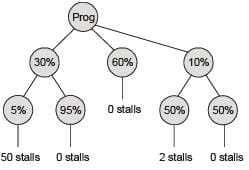Number of stalls/instruction = 0.85
Average instruction ETpipe = (1 + Number of stalls/instruction) × Cycle time
= (1 + 0.85) × 0.5 ns = 0.925 ns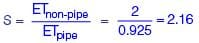Test : CSE Past Year Paper 2020 - Question 44

Which of the following  languages are undecidable?  Note that 〈 M〉 indicates encoding of the Turing machine M.
L1 = {〈M〉⏐L(M) = φ]
L2 = {〈M, w, q〉⏐M on input w reaches state q in exactly 100 steps}
L3 = {〈M〉⏐L(M) is not recursive)
L4 = {〈M〉⏐L(M) contains at least 21 members)

Detailed Solution for Test : CSE Past Year Paper 2020 - Question 44

Rice’s theorem applies to L1, L3 and L4 since then have condition based on L(M), which are non-trivial.
L1 : L(M)= φ is non-trivial condition because some Turing machines accept φ and some don’t.
L3 : L(M)= non-recursive, is non-trivial because some Turing machines accept nonrecursive and some accept recursive.
L4 : ⏐L(M)⏐ ≥ 21 is non-trivial since some Turing machines accept more than 21 strings, some accept less.
L2 : We cannot apply Rice’s theorem since condition is not on L(M) but on M reaching a state q in exactly 100 steps which can be checked on a UTM.
So L2 is decidable.
So, option (b), L1, L3 and L4 are undecidable is correct.

Test : CSE Past Year Paper 2020 - Question 45

Consider a schedule of transactions T1 and T2: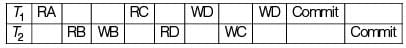Here, RX stands for “Read(X)” and WX stands for “Write(X)'”. Which one of the following schedules is conflict equivalent to the above schedule?

Detailed Solution for Test : CSE Past Year Paper 2020 - Question 45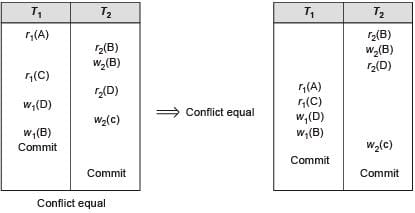Test : CSE Past Year Paper 2020 - Question 46

Which one of the following predicate formulae is NOT logically valid?
Note that W is a predicate formula without any free occurrence of x.

Detailed Solution for Test : CSE Past Year Paper 2020 - Question 46

∀x (p (x) → W ) ⇒ (∀x p (x)) → W is true.
But (∀x p (x)) → W ⇒ ∀x (p (x) → W ) is false.
Since if LHS is true then (P1 ∧ P2 ∧ P3 ... ∧ Pn) → W will be true and RHS will be false since P1 → W , itself will be false, since only P1 true cannot make W true (we need all the P1, P2 ..., Pn to be true to make W true)
So LHS ⇒ RHS true and RHS ⇒ LHS is false so LHS ≠ RHS.
So option (b) is invalid.
Note that option (c) is true because by Boolean algebra,
LHS = ∃x (p (x) → W ) ≡ ∃x (∼p (x) ∨ W )
≡∃x (∼p (x)) ∨ W
RHS = ∀x p (x) → W ≡ ∼ (∀x p (x)) ∨ W
≡∃x ∼p (x) ∨ W
So LHS = RHS.

Test : CSE Past Year Paper 2020 - Question 47

In a balanced binary search tree with n elements, what is the worst case time complexity of reporting all elements in range [a, b]? Assume that the number of reported elements is k.

Detailed Solution for Test : CSE Past Year Paper 2020 - Question 47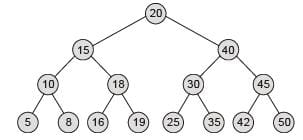Let a = 16, b = 42
16: Find the ‘16’ element in the BST = Ο(logn)
42: Find the ‘42’ element in the BST = Ο(logn)
[16, 42]: Inorder sorted element between 16 to 42
{16, 18, 19, 20, 25, 30, 35, 40, 42} ⇒ requires Ο(k) time for k element
So total time: Ο(2 logn + k) ≡ Ο(logn + k)

*Answer can only contain numeric values
Test : CSE Past Year Paper 2020 - Question 48

Consider the following C functions: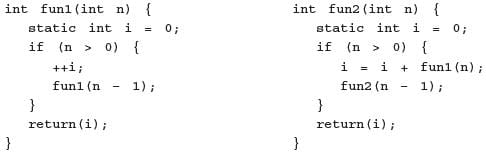The return value of fun2 (5) is ________.

Detailed Solution for Test : CSE Past Year Paper 2020 - Question 48

• Variable i is static in both the functions thus its value will persist throughout the program.
• When fun2 (5) gets called, it will first call fun1 with value of n as 5 and then fun2 will gets called with n = 4.
• Similarly, same steps are taken for all the upcoming values of n.

Test : CSE Past Year Paper 2020 - Question 49

Consider the productions A → PQ and A → XY. Each of the five non-terminals A, P, Q, X and Y has two attributes: s is a synthesized attribute, and i is an inherited attribute.
Consider the following rules.
Rule 1: P.i = A.i + 2, Q.i = P.i + A.i and A.s = P.s + Q.s
Rule 2: X.i = A.i + Y.s and Y.i = X.s + A.i
Which one of the following is TRUE?

Detailed Solution for Test : CSE Past Year Paper 2020 - Question 49

Rule 1: It is L-attributed by definition.
Rule 2: It is not L-attributed because this expression (X.i = A.i + Y.s) fails to satisfy the definition of L-attributed.

*Answer can only contain numeric values
Test : CSE Past Year Paper 2020 - Question 50

For n > 2, let  a ∈ {0,1}n be a  non-zero vector. Suppose that x is chosen uniformly at random from {0, 1}n. Then, the probability that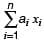is an odd number is ________.

Detailed Solution for Test : CSE Past Year Paper 2020 - Question 50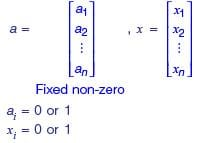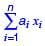value lies between 0 to n .
Total number cases = 2n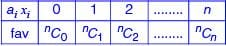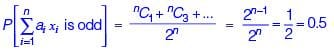*Answer can only contain numeric values
Test : CSE Past Year Paper 2020 - Question 51

Consider a database implemented using B+ tree for file indexing and installed on a disk drive with block size of 4 KB. The size of search key is 12 bytes and the size of tree/ disk pointer is 8 bytes. Assume that the database has one million records. Also assume that no node of the B+ tree and no records are present initially in main memory. Consider that each record fits into one disk block. The minimum number of disk accesses required to retrieve any record in the database is ________.

Detailed Solution for Test : CSE Past Year Paper 2020 - Question 51

Block size = 4 KB
Search key = 12 bytes
Tree pointer = 8 bytes
DB records 1 million = 1000000
B+ tree index each record fits into one block
⇒ Keys given to find levels of B+ tree : Bulk loading B+ tree design we can use
⇒ Order of B tree nodes P × Bp + (P – 1) Keys ≤ Block size
P × 8 + (P – 1)12 ≤ 4096
20P ≤ 4108
P = [4108/20] = 205
⇒ Minimum levels and index: Max fill factor of each node.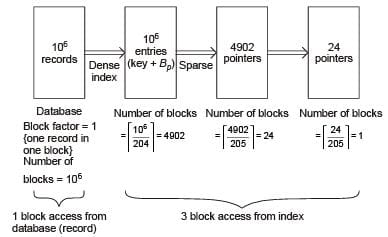⇒ Minimum 4 block access required to access record.

*Answer can only contain numeric values
Test : CSE Past Year Paper 2020 - Question 52

The number of permutations of the characters in LILAC so that no character appears in its original position, if the two L’s are indistinguishable, is ________.

Detailed Solution for Test : CSE Past Year Paper 2020 - Question 52

Since both L’s are indistinguishable.
First L’s can be arranged in 3 positions 2, 3, or 5 in 3C2 = 3 ways as follows: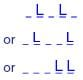Now the letters I, A, C can be deranged in 2 × 2! ways. Example in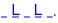C cannot occupy 5th position, so only 2 ways.
Remaining I and A can be arranged in remaining 2 position in 2! ways = 2 ways.
So answer is 3 × 2 × 2! = 12.

*Answer can only contain numeric values
Test : CSE Past Year Paper 2020 - Question 53

Graph G is obtained by adding vertex s to K3, 4 and making s adjacent to every vertex of K3, 4. The minimum number of colours required to edge-colour G is ________.

Detailed Solution for Test : CSE Past Year Paper 2020 - Question 53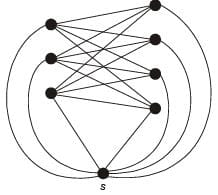7 edges are touching at S each of which needs to be colors by different color.
So 7 colors minimum are required. All other vertices have less than 7 edges touching.
So 7 colors only are required for edge coloring.

Test : CSE Past Year Paper 2020 - Question 54

Consider the Boolean function z (a, b, c ).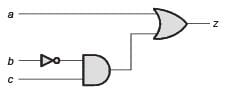Which one of the following minterm lists represents the circuit given above?

Detailed Solution for Test : CSE Past Year Paper 2020 - Question 54

∴ z (a , b, c)=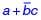K-map of the output z is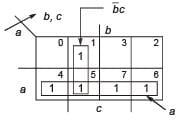∴ z (a , b , c)= ∑m (1, 4, 5, 6, 7)

Test : CSE Past Year Paper 2020 - Question 55

A computer system with a word length of 32 bits has a 16 MB byte-addressable main memory and a 64 KB, 4-way set associative cache memory with a block size of 256 bytes. Consider the following tour physical addresses represented in hexadecimal notation.
A1 = 0x42C8A4, A2 = 0x546888, A3 = 0x6A289C, A4 = 0x5E4880
Which one of the following is TRUE?

Detailed Solution for Test : CSE Past Year Paper 2020 - Question 55

Word length = 32 bit, MM size = 16 MB
Physical address = 24 bit, CM size = 64 KB
4-way set associative
Block size = 256 B
Number of lines (N)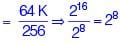Number of sets (S) =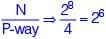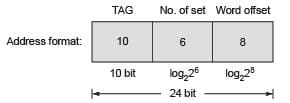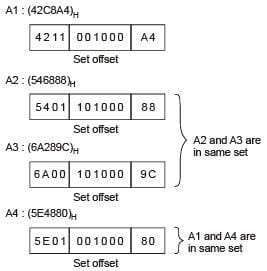Hence, option (b) is correct choice.

*Answer can only contain numeric values
Test : CSE Past Year Paper 2020 - Question 56

Consider a graph G = (V , E ), where V = {v2, v2, ..., v100}, E = {(vi , vj)⏐1 ≤ i < j ≤ 100} and weight of the edge (vi, vj) is ⏐i – j⏐. The weight of minimum spanning tree of G is ________.

Detailed Solution for Test : CSE Past Year Paper 2020 - Question 56

Consider small instance of 4-vertices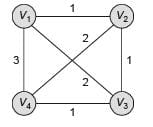Clearly,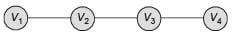would be a MST with cost 3.
Hence for 100 vertices, 99 will the weight of minimum spanning tree.

Test : CSE Past Year Paper 2020 - Question 57

Let G = (V, E ) be a directed, weighted graph with weight function w : E → R. For some function f : V → R, for each edge (u , v ) ∈ E, define w ′(u , v ) as w (u , v ) + f (v ).
Which one of the options completes the following sentence so that it is TRUE?
“The shortest paths in G under w are shortest paths under w′ too, ________”.

Detailed Solution for Test : CSE Past Year Paper 2020 - Question 57

w (u, v )= (u , v ) edge weight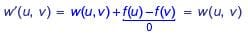So, option (a) correct.

Test : CSE Past Year Paper 2020 - Question 58

Let A and B be two n × n matrices over real numbers. Let rank(M) and det(M) denote the rank and determinant of a matrix M, respectively. Consider the following statements:
I. rank(AB) = rank(A) rank(B)
II. det(AB) = det(A) det(B)
III. rank(A + B) ≤ rank(A) + rank(B)
IV. det(A + B) ≤ det(A) + det(B)
Which of the above statements are TRUE?

Detailed Solution for Test : CSE Past Year Paper 2020 - Question 58

Statement II and III are correct statements directly based on properties of matrices.

Test : CSE Past Year Paper 2020 - Question 59

Consider three registers R1, R2, and R3 that store numbers in IEEE-754 single precision floating point format. Assume that R1 and R2 contain the values (in hexadecimal notation) 0x42200000 and 0xC1200000, respectively.
If R3 = R1/R2, what is the value stored in R3?

Detailed Solution for Test : CSE Past Year Paper 2020 - Question 59

R1: 0x42200000
R2: 0xC1200000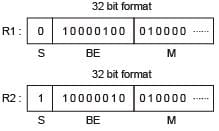1. Subtract the exponents i.e. divisor exponent from the dividend exponent.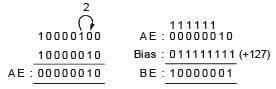2. Divide the mantissa i.e.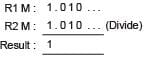3. Sign (opposite sign division result sign is –ve)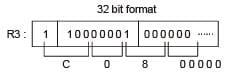Hence, option (a) is correct answer.

Test : CSE Past Year Paper 2020 - Question 60

Consider the following languages.
L1 = {w xy x ⏐w, x , y ∈ (0 + 1)+}
L2 = {xy ⏐x , y ∈ (a + b)*, ⏐x ⏐=⏐y ⏐, x ≠ y}
Which one of the following is TRUE?

Detailed Solution for Test : CSE Past Year Paper 2020 - Question 60

L1 = {w xyz ⏐w, x , y ∈ (0 + 1)+} is regular because by putting x as “0” and then “1” we can create a subset w 0 y 0 + w 1y1
r = (0 + 1)+ 0 (0 + 1)+ 0 + (0 + 1)+ 1(0 + 1)+ 1
Which can be shown to cover all other strings obtained by putting x as “00”, “01”, “10” and “11” etc.
Hence we can write regular expression r to represent given language “L1” and hence it is regular.
L2 has strings which can be broken into two parts x and y with not only equal length but also x ≠ y . The x ≠ y involves, string matching which FA cannot do and hence L2 is not regular.
It is however, context free because even length strings always have ⏐x⏐=⏐y⏐. So only one strings matching required and hence CFL.

*Answer can only contain numeric values
Test : CSE Past Year Paper 2020 - Question 61

Consider the following set of processes, assumed to have arrived at time 0. Consider the CPU scheduling algorithms Shortest Job First (SJF) and Round Robin (RR). For RR, assume that the processes are scheduled in the order P1, P2, P3, P4.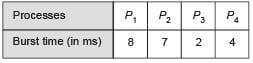If the time quantum for RR is 4 ms, then the absolute value of the difference between the average turnaround times (in ms) of SJF and RR (round off to 2 decimal places) is ________.

Detailed Solution for Test : CSE Past Year Paper 2020 - Question 61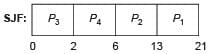Turn Around Time (TAT) = (21 – 0) + (13 – 0) + (2 – 0) + (6 – 0)
Average TAT = 42/4 = 10.5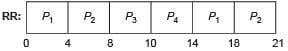Turn Around Time (TAT) = (18 – 0) + (21 – 0) + (10 – 0) + (14 – 0)
= 18 + 21 + 10 + 14
Average TA = 63/4 = 15.75
Hence, ⏐SJF (TAT) – RR(TAT)⏐
= ⏐10.5 – 15.75⏐ = 5.25

*Answer can only contain numeric values
Test : CSE Past Year Paper 2020 - Question 62

Consider a  TCP connection between a client and a server with the following specifications: the round trip time is 6 ns, the size of the receiver advertised window is 50 KB, slow start threshold at the client is 32 KB, and the maximum segment size is 2 KB. The connection is established at time t = 0. Assume that there are no timeouts and errors during transmission. Then the size of the congestion window (in KB) at time t + 60 ms after all acknowledgments are processed is ________.

Detailed Solution for Test : CSE Past Year Paper 2020 - Question 62

At time t = 0 there are not timeouts
Threshold = 32 KB
MSS = 2 KB
At time t + 60, window size = 44

*Answer can only contain numeric values
Test : CSE Past Year Paper 2020 - Question 63

A processor has 64 registers and uses 16-bit instruction format. It has two types of instructions: I-type and R-type. Each I-type instruction contains an opcode, a register name, and a 4-bit immediate value. Each R-type instruction contains an opcode and two register names. If there are 8 distinct I-type opcodes, then the maximum number of distinct R-type opcodes is ________.

Detailed Solution for Test : CSE Past Year Paper 2020 - Question 63

Given, 16 bit instruction and 64 registers
I-type: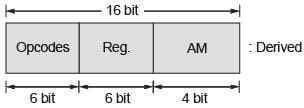R-type: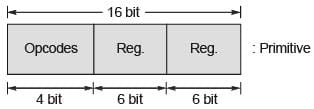1. Primitive instruction
R-type: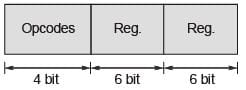2. Number of operation possible = 24 = 16
3. Number of tree opcodes = (16 – x)
Assume x is number of R-type instruction existed.
4. Number of I-type instruction possible = (16 – x) × 22
8 = 64 – 4 x
4x = 64 – 8 = 56
x = 56/4 ⇒ 14

Test : CSE Past Year Paper 2020 - Question 64

An organization requires a range of IP addresses to assign one to each of its 1500 computers. The organization has approached an Internet Service Provider (ISP) for this task. The ISP uses ClDR and serves the requests from the available IP address space 202.61.0.0/17. The ISP wants to assign an address space to the organization which will minimize the number of routing entries in the ISP's router using route aggregation.
Which of the following address spaces are potential candidates from which the ISP can allot any one to the organization?
I. 202.61.84.0/21
II. 202.61.104.0/21
III. 202.61.64.0/21
IV. 202.61.144.0/21

Detailed Solution for Test : CSE Past Year Paper 2020 - Question 64

/17 ⇒ 11111111 11111111 10000000 00000000
⇒ 255.255.128.0
1500 ≈ 2048 = 211 = 23 × 28
=232 – 21 = 211 = /21
/17 ⇒ 202.61.0.0/17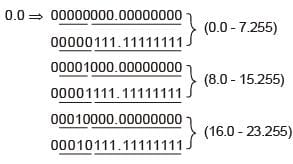Sequence is 0, 8, 16, 24, 32, 40, 48, 56, 64, 72, 80, 96, 104, 112, 120.
Hence 64 and 104 is present in sequence so it is the possible IP addresses.
So option (d) is correct.

Test : CSE Past Year Paper 2020 - Question 65

Consider a relational table R that is in 3NF, but not in BCNF. Which one of the following statements is TRUE?

Detailed Solution for Test : CSE Past Year Paper 2020 - Question 65

R(A, B, C, D, E)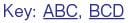FD: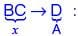‘x’ is not super key and ‘A’ is prime attribute.

 Use Code STAYHOME200 and get INR 200 additional OFF Use Coupon Code
Information about Test : CSE Past Year Paper 2020 Page
In this test you can find the Exam questions for Test : CSE Past Year Paper 2020 solved & explained in the simplest way possible. Besides giving Questions and answers for Test : CSE Past Year Paper 2020, EduRev gives you an ample number of Online tests for practice